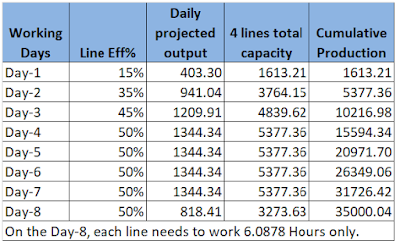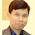# Calculate No. of Days Needed to Complete an Order of 35000 Pieces

Normally, garment factories prepare line loading plan in advance. The line loading planning is done based on the projected daily production capacity of a line. In this post, we will see the method of calculating the number of days needed for completing an order of 35000 pieces. I have also explained the formula for calculating the production capacity of a line.

The problem and the line performance details are given below. (The Problem is taken from a Facebook post)

 Order Quantity: 35000 pieces Daily Shift: 10 Hours Garment SMV: 26.5 Manpower: 125 per line Number of lines: 4   Line Eff: 50% and Learning curve: Day-1:15%, Day-2: 35% and Day-3:45%  Day-4 onwards:50% Absenteeism: 5% (Attendance: 95%)

First, let me explain the capacity calculation formula with absenteeism:
= (Daily Available minutes / Product SMV)
= (Daily Shift hours x 60 x Manpower x Line Eff% x Employee attendance %)/ Product SMV

In the first day of loading projected production capacity (when line Efficiency =15%)
= (10 x 60 x 125 x 15% x 95%)/26.50
= 403.30 pieces

In Day-2 production capacity (line efficiency 35%)
= (10 x 60 x 125 x 35% x 95%)/26.50
= 941.04 pieces

In Day-3 production capacity (line efficiency 45%)
= (10 x 60 x 125 x 45% x 95%)/26.50
= 1209.91 pieces

Day-4 onward projected line capacity will be (line efficiency 50%)
In Day-3 production capacity
= (10 x 60 x 125 x 50% x 95%)/26.50
= 1344.34 pieces

Day-wise total production capacity from 4 lines and cumulative production is shown in the following table. In the last day, lines don't need to work 10 hours to stitch the balance quantity.Table: Daily capacity calculation
In the above chart, you can see till Day-7, the total projected production volume is 31736.42 pieces. To reach 35,000 pieces you need to produce 3273.63 pieces on 8th day of production. PRoduction needed from each line is 818.41 pieces. So, I did reverse calculation to find how many hours required to make 818.41 pieces per line. It is for 6.0878 hours.

From the above calculations, 4 lines need to work for 8 days to produce 35,000 pieces of an order.

Related Post: How to calculate the production capacity of a garment factory?

1.In the above calculations, there is a slight error. In place of 6, it should read as 60. Kindly correct

1.Hi Vivek, thank you for finding and informing the mistakes. Correction is done.

2.Why do you multiply in 60, where did you get it?

3.Hi, 60 is multiplied in the above calculations to convert hours into minutes. Factory works 10 hours a day, which is equal to 600 minutes. Reason, the product SMV is in minutes. We are dividing the total available minutes by garment SMV to calculate production capacity.

2.I am interested on calculation the cutting room production capacity in minutes, separatly for all cutting tools and the number of workers .